# Semi-invariant(2)

(diff) ← Older revision | Latest revision (diff) | Newer revision → (diff)

A common eigenvector of a family of endomorphisms of a vector space or module. Ifis a set of linear mappings of a vector spaceover a field, a semi-invariant ofis a vector,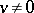, such thatwhereis a function, called the weight of the semi-invariant. A semi-invariant of weightis also called an invariant. The most frequently considered case is that of a linear group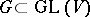, in which caseis a character ofand may be extended to a polynomial function on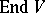. If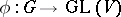is a linear representation of a groupin, then a semi-invariant of the groupis also called a semi-invariant of the representation(cf. also Linear representation, invariant of a). Letbe a linear algebraic group,a closed subgroup ofand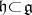the Lie algebras of these groups. Then there exist a faithful rational linear representationand a semi-invariant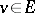ofsuch thatandare the maximal subsets ofandwhose images inhaveas semi-invariant. This implies that the mapping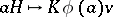,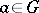, defines an isomorphism of the algebraic homogeneous space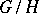onto the orbit of the straight line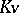in the projective space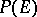.

The term semi-invariant of a setis sometimes applied to a polynomial function onwhich is a semi-invariant of the set of linear mappingsof the space, whereIfis a linear algebraic group andis its Lie algebra, thenhas semi-invariants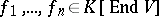of the same weight such thatandare the maximal subsets of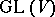andfor which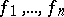are semi-invariants (Chevalley's theorem).

How to Cite This Entry:
Semi-invariant(2). Encyclopedia of Mathematics. URL: http://encyclopediaofmath.org/index.php?title=Semi-invariant(2)&oldid=12017
This article was adapted from an original article by A.L. Onishchik (originator), which appeared in Encyclopedia of Mathematics - ISBN 1402006098. See original article